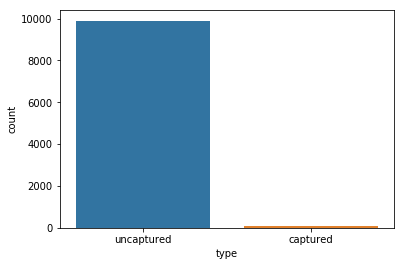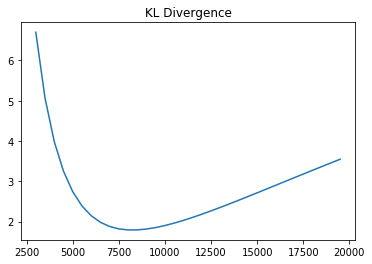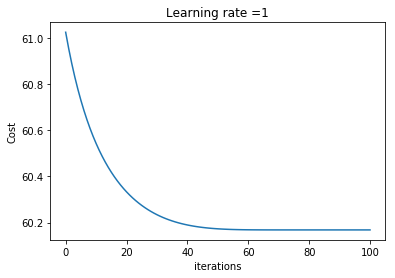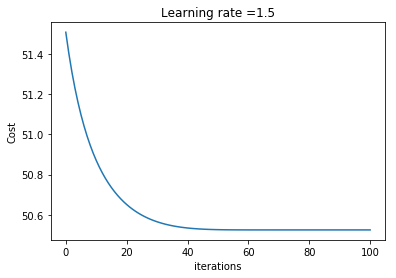# The KL Divergence¶

### Elan Ding¶

Modified: Aug. 4, 2018 $\newcommand{\bs}{\boldsymbol}$ $\newcommand{\argmin}{\underset{\bs{#1}}{\text{arg min}}\,}$ $\newcommand{\argmax}{\underset{\bs{#1}}{\text{arg max}}\,}$ $\newcommand{\tr}{^{\top}}$ $\newcommand{\norm}{\left|\left|\,#1\,\right|\right|}$ $\newcommand{\given}{\,|\,}$

## 1. Introduction¶

In this post I would like to explore a statistical method called capture-recapture. I have been pondering about this recently in relation to my previous post, in which I presented my favorite quote about statistics:

Statistics is concerned with estimating the unknown data-generating distribution $p(\bs{x})$ using the empirical distribution from the observed data $\widehat{p}(\bs{x})$. We then come up with our modeled distribution in a parametric family $p_{\text{model}}(\bs{x}; \theta)$ indexed by the parameter $\theta$.

This quote has prompted me to think about many previously-learned topics in a different light.Imagine that there is a pond full of fish, and our task is to estimate the size of the population. (In the rest of the post, I will refer to this as the fish-pond-problem.) We can use the capture-recapture technique, which starts out as follows.

1. First we capture $R$ fish from the pond, mark them, and release them back into the pond.
2. In a few days, we recapture $n$ fish, and note that $x$ of them are marked.
3. Based on $x$, we can estimate the total population in the pond.

Thinking like a statistician, the first step towards solving a problem is always coming up with a probabilistic interpretation. Recall that in linear regression, we assumed that $y = \theta\tr \bs{x} + \varepsilon$, where the error $\varepsilon$ follows a normal distribution centered at 0. Unlike regressional analysis, the fish-pond-problem provides no explicit output, but we can easily define it as the number of marked fish obtained from the recapture.

Hence, the unknown data-generating distribution $p(x)$ is the probability distribution of $x$ where $x$ is the true number of fish one would obtain from the recapture. The empirical distribution $\widehat{p}(x)$ is not very interesting if there is only one recapture. However, if feasible, one can perform the recapture many times, and obtain a whole spectrum of values for $x$. This forms the empirical distribution! Let's do a brief simulation of the scenario.

### Step 1 - Capture¶

We are going to simulate a pond of 10,000 fish, out of which 100 are marked.

In :
import numpy as np
import matplotlib.pyplot as plt
import pandas as pd
import seaborn as sns
%matplotlib inline

In :
# Give birth to 10000 fish; 100 are captured and marked
uncaptured = np.zeros(9900)
captured = np.ones(100)
pond = np.hstack([uncaptured.ravel(), captured.ravel()]).reshape(10000,1)

# Randomize the order
np.random.seed(18)
np.random.shuffle(pond)

# Show a bar graph
df = pd.DataFrame(pond, columns=['type'])
df['type'] = df['type'].apply(lambda x: 'captured' if x == 1 else 'uncaptured')
sns.countplot(x='type', data=df)

Out:
<matplotlib.axes._subplots.AxesSubplot at 0x11752a9b0>### Step 2 - Recapture¶

Since the pond is already randomized, we can select the first 500 in the pond as the recaptured fish and count the number of marked fish. (I assume that counting the number of marked fish is much easier than marking the fish; hence we can afford to use a larger sample size.)

In :
recaptured = pond[:500]
unique, counts = np.unique(recaptured, return_counts=True)
print('Among the 500 recaptured fish, {} are marked'.format(counts))

Among the 500 recaptured fish, 6 are marked


### Step 3 - Estimation¶

Let $X$ denote the total number of fish in the pond. There are many ways to estimate the total population. Using the simple proportions method from kindergarten, it is reasonable to say that

$$\frac{X}{100} = \frac{500}{6} \quad \implies \quad X \approx 8333$$

From a practical point of view, we are done here. The justification behind the proportions method is that we are using the sample proportion as a point estimate for the population proportion. But as mathematicians, we certainly want to go deeper. For example, what if we have more than one samples collected?

## 2. Modeled Distribution¶

Recall an additional fact from my previous post about the KL divergence:

Definition. The KL divergence between the empirical distribution $\widehat{p}$ and the model distribution $p_{\text{model}}$ is defined by

$$D_{\text{KL}}(\widehat{p}, p_{\text{model}}) = E_{\bs{x}\sim \widehat{p}} \left[\log \widehat{p}(\bs{x})-\log p_{\text{model}}(\bs{x}; \theta) \right]$$

As mentioned earlier, the empirical distribution is simply $\widehat{p}(6)=1$. It is up to us to decide what the modeled distribution should be. In the fish-pond-problem, it is obvious that the true distribution $p(x)$ follows a hypergeometric distribution. Hence, without considering computational costs, it is ideal to use the following probability mass function

$$p_{\text{model}}(x ; W) = \frac{\binom{100}{x}\binom{W}{500-x}}{\binom{100+W}{500}},$$

where $W$ is the number of unmarked fish in the pond.

Now we have everything we need to compute the KL divergence:

\begin{aligned} D_{\text{KL}}(\widehat{p}, p_{\text{model}}) &= \log \widehat{p}(6) - \log p_{\text{model}}(6) \\ &= -\log \frac{\binom{100}{6}\binom{W}{500-6}}{\binom{100+W}{500}} \\ &= -\log\left[\left(\frac{100! 500!}{6! 94! 494!}\right) \left(\frac{W!}{(W-494)!}\right) \left( \frac{(W-400)!}{(W+100)!} \right) \right] \end{aligned} \tag{1}

Taking log is the best way to neuralize the expoding behavior of the factorial. If we were to compute the binomial coefficients separately, we will easily run into the overflow problem. Just to give you an idea, the binomial coefficient $C(100, 24)$ is around $8 \times 10^{22}$. And we are dealing with $W \approx 9900$! So it is much better to take the log first and then sum them. Hence we define the logsum function.

In :
def logsum(end, start=1):
s = 0
for i in range(int(start), int(end)+1):
s += np.log(i)
return s


The constant term $\log (100! 500!)/(6! 94! 494!)$ in (1) can be ignored since it does not affect the minimization process, but for the sake of finding the exact value of the KL divergence, it can be easiliy computed as:

In :
logsum(100)+logsum(500)-logsum(6)-logsum(94)-logsum(494)

Out:
58.156480350943184

So the KL divergence can be implemented as follows.

In :
def KL_divergence(W):
a = logsum(W, W-493) - logsum(W+100, W-399)
return -a-58.156480350943184

# Vectorize the expression for convenience
KL_divergence_vectorized = np.vectorize(KL_divergence)

In :
import matplotlib.pyplot as plt
x = np.arange(3000,20000,500)
y = KL_divergence_vectorized(x)
plt.plot(x,y)
plt.title('KL Divergence')

Out:
Text(0.5,1,'KL Divergence')We see that the KL divergence has a global minimum at around 8000. How do we find the exact value of the minimum? Let's use a numerical optimization method called Nelder-Mead Method.

In :
from scipy.optimize import minimize

Out:
 final_simplex: (array([[8233.59375   ],
[8233.59367847]]), array([1.79177562, 1.79177562]))
fun: 1.7917756168003507
message: 'Optimization terminated successfully.'
nfev: 69
nit: 28
status: 0
success: True
x: array([8233.59375])

We see that $W\approx 8234$ minimizes the KL divergence. This is fairly close to the previously estimated value of $8333$.

## 3. Binomial Approximation¶

Imagine there is a bag containing $R+W$ balls, $R$ of which are red balls, and $W$ are white balls. In a single draw of $n$ balls from the bag without replacement, denote by $X$ the number of red balls drawn. Then, $X$ follows a hypergeometric distribution with parameters $R, W, n$. The probability mass function of $X$ is given by

$$P(X=x) = \frac{\binom{R}{x}\binom{W}{n-x}}{\binom{R+W}{n}}.$$

If $n$ very small compared to $R+W$, like in the fish-tank-problem where $n=500$ and $R+W=10,000$, the hypergeometric distribution can be approximated by a binomial distribution with parameters $n$ and $R/(R+W)$. This should be clear intuitively, as the draws become more dependent as the number of draws become large. Using this approximation, our modeled distribution can be written as

$$p_{\text{model}}(x ; W) = \binom{500}{x} \, \left(\frac{100}{100+W}\right)^x \, \left(\frac{W}{100+W}\right)^{500-x}$$

The KL divergence can be computed as

\begin{aligned} D_{\text{KL}}(\widehat{p}, p_{\text{model}}) &= \log \widehat{p}(6) - \log p_{\text{model}}(6; W) \\ &= -\log \binom{500}{6} \, \left(\frac{100}{100+W}\right)^6 \, \left(\frac{W}{100+W}\right)^{494} \\ &\propto - 494 \log W + 500 \log(100+W) \end{aligned} \tag{2}

where the sign $\propto$ means proportional to, after removing all the constants that do not affect the optimization process. Let's optimize this function using TensorFlow, with gradient descent optimization.

In :
import tensorflow as tf

In :
# Define our optimization model
def minimize_cost(cost_func, learning_rate=1):

tf.reset_default_graph()
costs = []

# Create a variable W that we are trying to optimize
W = tf.Variable(5000, dtype="float64", trainable=True)

# The loss function here is proportional to the KL divergence in (2)
Cost = cost_func(W)

init = tf.global_variables_initializer()

# Executing the computational graph
with tf.Session() as sess:
sess.run(init)
for i in range(10001):
W_value, Cost_value = sess.run([W,Cost])
if i % 1000 == 0:
print ("Cost after iteration %i: %f; The value of W: %f" % (i, Cost_value, W_value))
if i % 100 == 0:
costs.append(Cost_value)
sess.run(opt)
print("Parameter has been trained!")

plt.plot(np.squeeze(costs))
plt.ylabel('Cost')
plt.xlabel('iterations')
plt.title("Learning rate =" + str(learning_rate))
plt.show()

parameter = sess.run(W)

return parameter

In :
# Define KL divergence loss
def binom_loss(W):
return 6*tf.log(W+100) + 50000/(W+100)

minimize_cost(binom_loss)

Cost after iteration 0: 61.025896; The value of W: 5000.000000
Cost after iteration 1000: 60.543325; The value of W: 5867.231959
Cost after iteration 2000: 60.333211; The value of W: 6549.205387
Cost after iteration 3000: 60.234089; The value of W: 7110.532913
Cost after iteration 4000: 60.189533; The value of W: 7567.093630
Cost after iteration 5000: 60.172868; The value of W: 7910.172693
Cost after iteration 6000: 60.168644; The value of W: 8123.418690
Cost after iteration 7000: 60.168130; The value of W: 8213.442346
Cost after iteration 8000: 60.168113; The value of W: 8232.141994
Cost after iteration 9000: 60.168113; The value of W: 8233.322762
Cost after iteration 10000: 60.168113; The value of W: 8233.333330
Parameter has been trained!Out:
8233.333330035855

I have to admit that using TensorFlow is overkill, but it is quite fun trying different optimization methods. We see that the minimum achieved is strikingly similar to the result obtained from using the hypergeometric distribution (8233.59).

## 4. Poisson Approximation¶

It is well known that a binomial distribution with parameters $n, p$, such that $n$ is much larger than $p$, can be approximated by a Poisson distribution with parameter $\lambda = np = 50,000/(100+W)$. Hence, we can further simplify our modeled distribution to have the following form.

$$p_{\text{model}}(x ; W) = \frac{\left(\frac{50,000}{W+100}\right)^x e^{-\frac{50,000}{W+100}} }{x!}.$$

The KL divergence becomes

\begin{aligned} D_{\text{KL}}(\widehat{p}, p_{\text{model}}) &= \log \widehat{p}(6) - \log p_{\text{model}}(6; W) \\ &= -\log \frac{\left(\frac{50,000}{W+100}\right)^6 e^{-\frac{50,000}{W+100}} }{6!} \\ &\propto 6\log(W+100) + \frac{50,000}{W+100} \end{aligned} \tag{3}

Let's try to minimize this function.

In :
# Define KL divergence loss
def poisson_loss(W):
return 6*tf.log(W+100) + 50000/(W+100)

minimize_cost(poisson_loss)

Cost after iteration 0: 61.025896; The value of W: 5000.000000
Cost after iteration 1000: 60.543325; The value of W: 5867.231959
Cost after iteration 2000: 60.333211; The value of W: 6549.205387
Cost after iteration 3000: 60.234089; The value of W: 7110.532913
Cost after iteration 4000: 60.189533; The value of W: 7567.093630
Cost after iteration 5000: 60.172868; The value of W: 7910.172693
Cost after iteration 6000: 60.168644; The value of W: 8123.418690
Cost after iteration 7000: 60.168130; The value of W: 8213.442346
Cost after iteration 8000: 60.168113; The value of W: 8232.141994
Cost after iteration 9000: 60.168113; The value of W: 8233.322762
Cost after iteration 10000: 60.168113; The value of W: 8233.333330
Parameter has been trained!Out:
8233.333330035855

The results are certainly very close! So which cost function do you prefer?

## 5. Sample Stratification¶

Suppose we break the sample $n=500$ into five samples of equal size.

In :
# Randomize the order 106
np.random.seed(122)
np.random.shuffle(pond)

recaptured_1 = pond[:100]
recaptured_2 = pond[100:200]
recaptured_3 = pond[200:300]
recaptured_4 = pond[300:400]
recaptured_5 = pond[400:500]

unique_1, counts_1 = np.unique(recaptured_1, return_counts=True)
unique_2, counts_2 = np.unique(recaptured_2, return_counts=True)
unique_3, counts_3 = np.unique(recaptured_3, return_counts=True)
unique_4, counts_4 = np.unique(recaptured_4, return_counts=True)
unique_5, counts_5 = np.unique(recaptured_5, return_counts=True)

print('Sample 1 [unmarked, marked]: {}'.format(counts_1))
print('Sample 2 [unmarked, marked]: {}'.format(counts_2))
print('Sample 3 [unmarked, marked]: {}'.format(counts_3))
print('Sample 4 [unmarked, marked]: {}'.format(counts_4))
print('Sample 5 [unmarked, marked]: {}'.format(counts_5))

Sample 1 [unmarked, marked]: [99  1]
Sample 2 [unmarked, marked]: [99  1]
Sample 3 [unmarked, marked]: [99  1]
Sample 4 [unmarked, marked]: [98  2]
Sample 5 [unmarked, marked]: [99  1]


In this new setup, the empirical distribution is defined by $\widehat{p}(1) = 4/5, \widehat{p}(2) = 1/5$. Using the Poisson modeled, we have:

$$p_{\text{model}}(x ; W) = \frac{\left(\frac{10,000}{W+100}\right)^x e^{-\frac{10,000}{W+100}} }{x!}.$$

The KL divergence becomes

\begin{aligned} D_{\text{KL}}(\widehat{p}, p_{\text{model}}) &\propto - E_{x\sim \widehat{p}} \log p_{\text{model}}(x; W) \\ &\propto - \frac{4}{5}\log \frac{\left(\frac{10,000}{W+100}\right)^1 e^{-\frac{10,000}{W+100}} }{1!} - \frac{1}{5}\log \frac{\left(\frac{10,000}{W+100}\right)^2 e^{-\frac{10,000}{W+100}} }{2!} \\ &\propto 4\frac{10,000}{W+100} + 4\log(W+100) + \frac{1}{2}\frac{10,000}{W+100} + \log(W+100) \\ &\propto 4.5 \frac{10,000}{W+100} + 5\log(W+100) \end{aligned} \tag{4}

Comparing (4) with (3), we see a slight difference. Let's use grandient descent to mimize it.

In :
def strata_loss(W):
return 5*tf.log(W+100) + 4.5*10000/(W+100)

minimize_cost(strata_loss, 1.5)

Cost after iteration 0: 51.508509; The value of W: 5000.000000
Cost after iteration 1000: 50.870813; The value of W: 6240.450845
Cost after iteration 2000: 50.651277; The value of W: 7146.423519
Cost after iteration 3000: 50.565380; The value of W: 7845.599261
Cost after iteration 4000: 50.534458; The value of W: 8365.690807
Cost after iteration 5000: 50.526165; The value of W: 8700.503189
Cost after iteration 6000: 50.524957; The value of W: 8856.870935
Cost after iteration 7000: 50.524900; The value of W: 8896.454420
Cost after iteration 8000: 50.524899; The value of W: 8899.945575
Cost after iteration 9000: 50.524899; The value of W: 8899.999955
Cost after iteration 10000: 50.524899; The value of W: 8900.000000
Parameter has been trained!Out:
8899.999999999814

Interesting! What happens here is that by adding more samples, the KL divergence method is doing slightly better than the simple porportions method since it is utilizing more information.